• 2KB qq_43436616 2020-03-31 10:43:08
•5星
53KB m0_52957036 2020-09-20 16:03:32
• 38KB m0_52957036 2020-10-08 09:27:33
• 4KB weixin_42028424 2019-01-17 14:25:34
• 3.82MB m0_52957036 2020-03-17 18:12:13
• 中值滤波代码 opencv

57.48MB wfh2015 2018-06-14 16:33:07
• 目录[OpenCV+VS2015]中值滤波、快速中值滤波和OpenCV中API中值滤波中值滤波快速中值滤波直方图快速中值滤波过程OpenCV中API总结： 中值滤波 简单来说就是用一个nn的框框（n为奇数）去框一个图像，框选中的nn个像素点...

[OpenCV+VS2015]中值滤波、快速中值滤波和OpenCV中API中值滤波

上课老师没有详细讲，自己查阅了一下然后做了点程序验证一下

中值滤波

简单来说就是用一个nn的框框（n为奇数）去框一个图像，框选中的nn个像素点中取中值，然后用中值取代替框框中间的那个点的像素
哎说起来简单，代码有点烧脑，直接撸上来

void MF(Mat& image_input, Mat& image_output, int diameter)
{
int  radius = (diameter - 1) / 2;//向下取整
int i, j, p, q, t,sum = 0,median;
uchar val;
int Hist = { 0 };
int Threshold = (diameter*diameter + 1) / 2;

for (i = 0; i < image_input.rows; i++)
{
for (j = 0; j <radius; j++)
{
image_output.at<uchar>(i, j) = image_input.at<uchar>(i, j);
image_output.at<uchar>(i, (image_input.cols - j - 1)) = image_input.at<uchar>(i, (image_input.cols - j - 1));
}
}
for (j = 0; j < image_input.cols; j++)
{
for (i = 0; i < radius; i++)
{
image_output.at<uchar>(i, j) = image_input.at<uchar>(i, j);
image_output.at<uchar>((image_input.rows - i - 1), j) = image_input.at<uchar>((image_input.rows - i - 1), j);
}
}
//处理完了边缘部分
{
{
memset(Hist, 0, sizeof(Hist));  //初始化直方图；
for (p = i - radius; p <= i + radius; p++)
{
for (q = j - radius; q <= j + radius; q++)
{
val = image_input.at<uchar>(p, q);
Hist[val]++;
}
}
for (t = 0; t < 256; t++)
{
sum = sum + Hist[t];
if (sum >= Threshold)
{
sum = 0;
median = t;
break;
}
}
//中值找到，需要替换
image_output.at<uchar>(i, j) = (uchar)median;
}
}
}

快速中值滤波

这里推荐一篇文献：A Fast Two-Dimensional Median Filtering Algorithm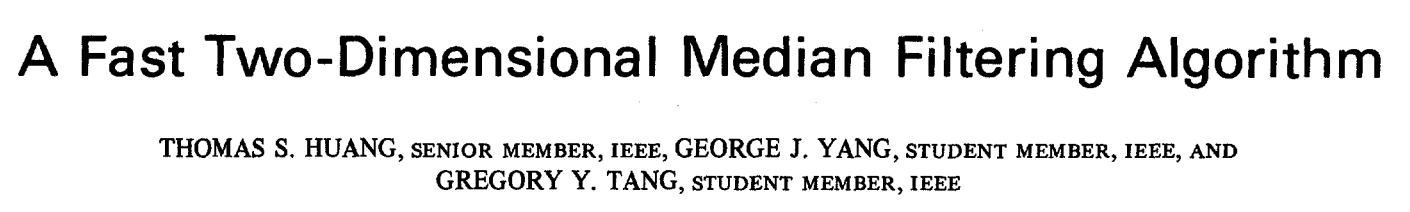这个讲的就是中值滤波是对每个可以滤波的点进行一遍中值计算，运算量还是比较大的。
但是，快速中值滤波简化了中值计算如图所示，对于同一行的像素点，其实它是向右平移一格，其实不需要计算所有像素点的中值：只要去掉原来的最左边一列，然后加上新的最右边一列，就可以形成新的直方图

直方图

这里用直方图的方法计算中值，对于灰度图来说一共有256级，可以新建一个数组作为直方图，当出现某一级灰度，变在这个直方图数组对应的灰度数组上加1；

快速中值滤波过程

一：建立第一个窗口的直方图，并找到中值。然后计算这个窗口里小于中值的像素个数作为itmdn
二：移动到下一个窗口，然后删掉最左边一列，加上最右边一列，然后更新灰度直方图，更新itmdn，这时候的itmdn存储的是当前窗口中小于上一个窗口中值的像素个数。
三：比较itmdn和(窗口像素数/2)，会有三种可能，
若itmdn大，中值左移；
若itmdn小，中值右移；
若一样大，中值不移动；
四：重复步骤二

上代码：

void  FMF(Mat& image_input, Mat& image_output,int diameter)  //用于提取边缘，保留不动
{
int i, j, p, q, s, t;
uchar val;
int sum = 0;
int Hist = { 0 };
int Threshold = (diameter*diameter + 1) / 2;
int median;

for (i = 0; i < image_input.rows; i++)
{
for (j = 0; j <radius; j++)
{
image_output.at<uchar>(i,j) = image_input.at<uchar>(i, j);
image_output.at<uchar>(i,(image_input.cols-j-1)) = image_input.at<uchar>(i, (image_input.cols - j - 1));
}
}
for (j = 0; j < image_input.cols; j++)
{
for (i = 0; i < radius; i++)
{
image_output.at<uchar>(i,j) = image_input.at<uchar>(i, j);
image_output.at<uchar>((image_input.rows-i-1),j) = image_input.at<uchar>((image_input.rows - i - 1), j);
}
}
//处理完了边缘部分

{
{
{
memset(Hist, 0, sizeof(Hist));  //初始化直方图；
for ( p = i - radius; p <=i + radius; p++)
{
for ( q = j - radius; q <= j + radius; q++)
{
val = image_input.at<uchar>(p, q);
Hist[val]++;
}
}
}//判断结束表示直方图建立完毕，后面要做的是更新直方图
{
for (s = i - radius; s <= i + radius; s++)
{
val = image_input.at<uchar>(s, j - radius - 1);
Hist[val]--;
val = image_input.at<uchar>(s, j + radius);
Hist[val]++;
}//直方图更新完毕
}
//寻找中值
for ( t = 0; t < 256; t++)
{
sum = sum + Hist[t];
if (sum >= Threshold)
{
sum = 0;
median = t;
break;
}
}
//中值找到，需要替换
image_output.at<uchar>(i, j) = (uchar)median;
}
}
}

OpenCV中API

简单的很，一句话搞定

medianBlur(src, dst, 9);

总结：

对外输出图片的中值滤波处理时间

Mat dst = Mat::zeros(src.size(),CV_8UC1);
start = clock();
FMF(src, dst, 9);
end = clock();
start = (end - start) / CLOCKS_PER_SEC;
printf("the fast filter consume time is %f s\n", start);
namedWindow("fast filter", WINDOW_AUTOSIZE);
imshow("fast filter", dst);
dst = Mat::zeros(src.size(), CV_8UC1);

上结果
当窗口直径为3时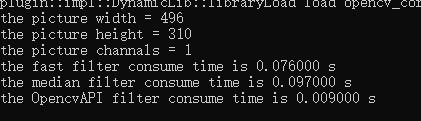当窗口直径为9时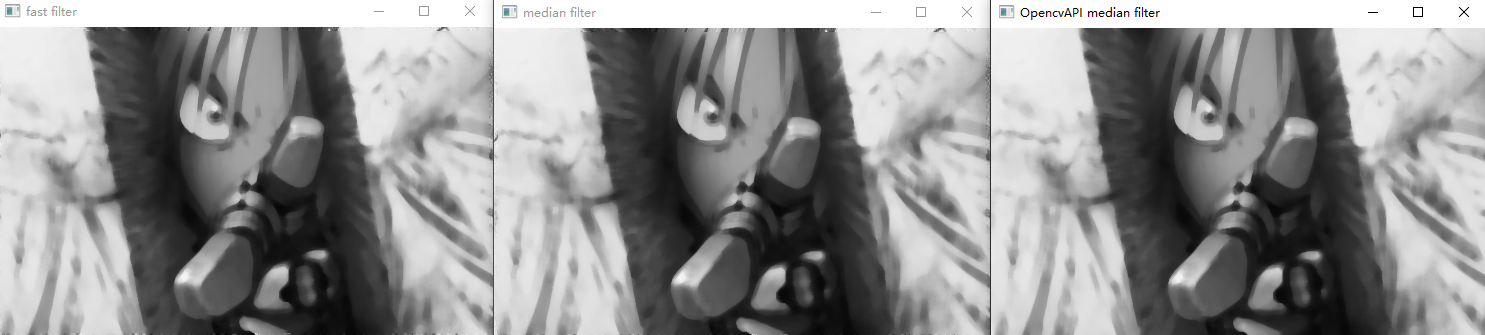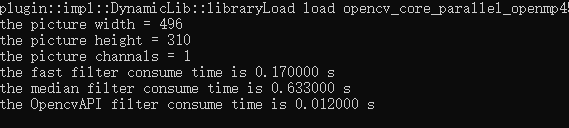当窗口仍然为9时，将图变大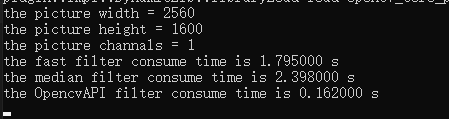所以啊：
1、快速中值滤波有一定的加快计算速度的效果，

2、而且随着窗口的变大，中值滤波和快速中值滤波所用的时间相差的越大，

3、当图片的大小越大，中值滤波和快速中值滤波所用的时间相差的越大（快速中值滤波比中值滤波快的更明显），

4、滤波窗口越大，图像越模糊，计算量越大

5、当然，都没有API快，hhhh

最后，钢炼yyds

展开全文weixin_49578216 2021-05-07 20:35:16
• NR基础篇下——中值滤波、多级中值滤波、多级中值混合滤波、加权中值滤波、中值有理滤波 1024程序员节 matlab 算法

中值滤波很好理解，均值滤波就是在一个小窗口中求均值来取代当前像素值，而中值滤波就是通过求小窗口中的中位值来取代当前位置的方式来滤波。 如图绿色窗口就是当前的滤波窗口，在一个3X3的邻域窗口中进行滤波。...

上一篇分享了一些均值滤波相关的算法，均值滤波作为一种线性滤波器，在滤除噪声的同时也会导致边缘模糊问题。而且均值滤波对高斯噪声的效果很好，但是对于椒盐噪声的效果就很一般。但是中值滤波作为一种顺序滤波器，对于椒盐噪声的效果很好，而且保边能力很强，所以这一篇主要讨论一下中值相关的算法。

中值滤波

算法原理

中值滤波很好理解，均值滤波就是在一个小窗口中求均值来取代当前像素值，而中值滤波就是通过求小窗口中的中位值来取代当前位置的方式来滤波。如图绿色窗口就是当前的滤波窗口，在一个3X3的邻域窗口中进行滤波。那么中值滤波做的就是：

1. 对这个邻域中的像素值进行排序：[45, 50, 52, 60, 75, 80, 90, 200, 255];
2. 从排序后的数据中找出中位值：75；
3. 用中位值取代当前位置的像素值，所以就可以得到右侧的滤波后的数据。

代码实现

%% --------------------------------
%% author:wtzhu
%% email:wtzhu_13@163.com
%% date: 20210306
%% fuction: 中值滤波
%% --------------------------------
clear;
clc;
close all;
[m, n] = size(img);
figure;subplot(221);imshow(img);title('original image');

%% 运算的时候需要对边缘进行扩展
% 需要特殊处理四周最外圈的行和列，本算法中将其向外扩展一圈，用最外圈的值填充
tailRowMat = img(m,:);%取f的第m行
% 行扩展后，列扩展时需要注意四个角需要单独扩展进去，不然就成了十字架形的
tailColumnMat = [img(1,n), img(:,n)', img(m,n)];
expandImage = uint8(expandImage');
subplot(222);imshow(expandImage);title('expand image');

newImg = zeros(m, n);
for i =2: m+1
for j =2: n+1
imgRoi = [expandImage(i-1, j-1) expandImage(i-1, j) expandImage(i-1, j+1) ...
expandImage(i  , j-1) expandImage(i  , j) expandImage(i  , j+1) ...
expandImage(i+1, j-1) expandImage(i+1, j) expandImage(i+1, j+1)];
orderedList = sort(imgRoi);
sizeRoi = size(imgRoi);
newImg(i-1, j-1) = orderedList((sizeRoi(2)+1)/2);
end
end
newImg = uint8(newImg);
subplot(223);imshow(newImg);title('new image');
subplot(224);imshow(uint8(newImg-img));title('newImg-img');从滤波效果图中可以看出去噪能力还可以，百块中的噪点去除了很多，而且边缘信息保留得也很好。

多级中值滤波

算法原理

简单的中值滤波器滤波效果有限，于是就有人提出了将多个中值滤波进行多级级联实现更好的滤波效果。如图就是一种多级级联的方式，先在窗口中定义一个’+'和’X’形的窗口，然后分别求出这两个窗口的中位值，然后结合当前窗口的中心点就有3个候选值，再从这三个值中求出一个中位值作为滤波后的结果。

这种方式也可以直接应用到RAW图中做BNR，需要修改的就是窗口设置为5X5，然后在做滤波的时候需要区分G和RB通道，因为这个前面已经讲过，RAW图中的RGB分布是不均匀的，G占50%，R和B各占25%。左侧就是针对G通道的滤波器，右侧是R和B通道的滤波器，都是定义了一个’+'和’X’形的窗口，不同的只是取的点的位置不同。

代码实现

%% --------------------------------
%% author:wtzhu
%% email:wtzhu_13@163.com
%% date: 20211023
%% fuction: multistage median filters
%% --------------------------------

close all;
clear all;
clc
I = double(img);
% I = double(imresize(img, [64, 64]));
figure();
imshow(uint8(I));
title('org file');

I_noise = I + 10 * randn(size(I));
figure();
imshow(uint8(I_noise));
title('noise file');
[m,n] = size(I_noise);

DenoisedImg = zeros(m,n);

tic
for i = 1: m
for j = 1: n
% first stage
median_HV = median([roi(1,2), roi(2,1), roi(2,2), roi(2,3), roi(3,2)]);
median_diag = median([roi(1,1), roi(1,3), roi(2,2), roi(3,1), roi(3,3)]);
% second stage
DenoisedImg(i, j) = median([median_HV, roi(2,2), median_diag]);
end
end
figure();
imshow(uint8(DenoisedImg));
title('denoise file');
toc

b = medfilt2(I_noise,[3,3]);
figure();
imshow(uint8(b));
title('median filter of matlab denoise file');

多级中值混合滤波

算法原理

前面介绍过中值滤波和均值滤波各自有各自的优点和缺点，所以就可以考虑将两者结合起来，相互弥补实现更好的滤波效果，于是就有人提出了这种多级混合滤波的方式。算法流程：

1. 求出竖直方向相邻三个点的均值和水平方向相邻三个点的均值，再结合当前点，用这三个点再求一个中位值；
2. 求出45°和135°方向上的均值，然后结合当前点求出一个中位值；
3. 两个中位值结合当前点组成新的数组，最后求一个中位值作为当前点的值完成滤波。

这样就巧妙的将均值滤波和中位值滤波结合了。然后如果应用在RAW图上，只需要对滤波器稍作改善即可。代码实现

%% --------------------------------
%% author:wtzhu
%% email:wtzhu_13@163.com
%% date: 20211023
%% fuction: multistage median hybird filters
%% --------------------------------

close all;
clear all;
clc
I = double(img);
figure();
imshow(uint8(I));
title('org file');

I_noise = I + 10 * randn(size(I));
figure();
imshow(uint8(I_noise));
title('noise file');
[m,n] = size(I_noise);

DenoisedImg = zeros(m,n);

tic
for i = 1: m
for j = 1: n
% first stage: average and median
mean_V = mean(roi(:,2));
mean_H = mean(roi(2,:));
median_HV = median([mean_V, roi(2, 2)], mean_H);

mean45 = mean([roi(1, 3), roi(2, 2), roi(3, 1)]);
mean135 = mean([roi(1, 1), roi(2, 2), roi(3, 3)]);
median_diag = median([mean45, roi(2, 2)], mean135);

% second stage
DenoisedImg(i, j) = median([median_HV, roi(2,2), median_diag]);
end
end
figure();
imshow(uint8(DenoisedImg));
title('denoise file');
toc

b = medfilt2(I_noise,[3,3]);
figure();
imshow(uint8(b));
title('median filter of matlab denoise file');

多级中值有理混合滤波

算法原理WMF加权中值滤波加权中值滤波也很好理解，和加权均值滤波差不多，就是在原始数据的基础上给每个点分别赋予一个权重，然后在加权后的数据中取出中位值作为滤波后的值。

算法流程

1. 求出’+'形和’X’形的窗口的中位值；

2. 对’+'形窗口再利用CWMF求出一个值，CWMF是WMF的一种特殊情况，就是只对中心点进行加权；

3. 对以上求出的三个参数用一下公式计算出一个新的值作为滤波后的值
y ( m , n ) = ϕ 2 ( m , n ) + ϕ 1 ( m , n ) − 2 ∗ ϕ 2 ( m , n ) + ϕ 3 ( m , n ) h + k ( ϕ 1 ( m , n ) − ϕ 3 ( m , n ) ) y(m, n)=\phi 2(m, n)+\frac{\phi 1(m, n)-2 * \phi 2(m, n)+\phi 3(m, n)}{h+k(\phi 1(m, n)-\phi 3(m, n))}

一样的，稍作改动该算法就可以用于raw格式图像代码实现

%% --------------------------------
%% author:wtzhu
%% email:wtzhu_13@163.com
%% date: 20211023
%% fuction: median rational hybird file filter
%% references: MEDIAN-RATIONAL HYBRID FILTERS
%% --------------------------------

close all;
clear all;
clc
I = double(img);
figure();
imshow(uint8(I));
title('org file');

I_noise = I + 10 * randn(size(I));
figure();
imshow(uint8(I_noise));
title('noise file');
[m,n] = size(I_noise);

DenoisedImg = zeros(m,n);

h = 2;
k = 0.01;

tic
for i = 1: m
for j = 1: n
median_HV = median([roi(1,2), roi(2,1), roi(2,2), roi(2,3), roi(3,2)]);
median_diag = median([roi(1,1), roi(1,3), roi(2,2), roi(3,1), roi(3,3)]);
CWMF = median([roi(1,2), roi(2,1), roi(2,2)*3, roi(2,3), roi(3,2)]);

DenoisedImg(i, j) = CWMF + (median_HV + median_diag - 2 * CWMF) / (h + k * (median_HV - median_diag));
end
end
toc
figure();
imshow(uint8(DenoisedImg));
title('denoise file');
b = medfilt2(I_noise,[3,3]);
figure();
imshow(uint8(b));
title('median filter of matlab denoise file');

相关链接

展开全文wtzhu_13 2021-10-24 18:54:45
• 数字图像处理 实验三 中值滤波处理 计算机视觉 图像处理 opencv

中值滤波

实验目的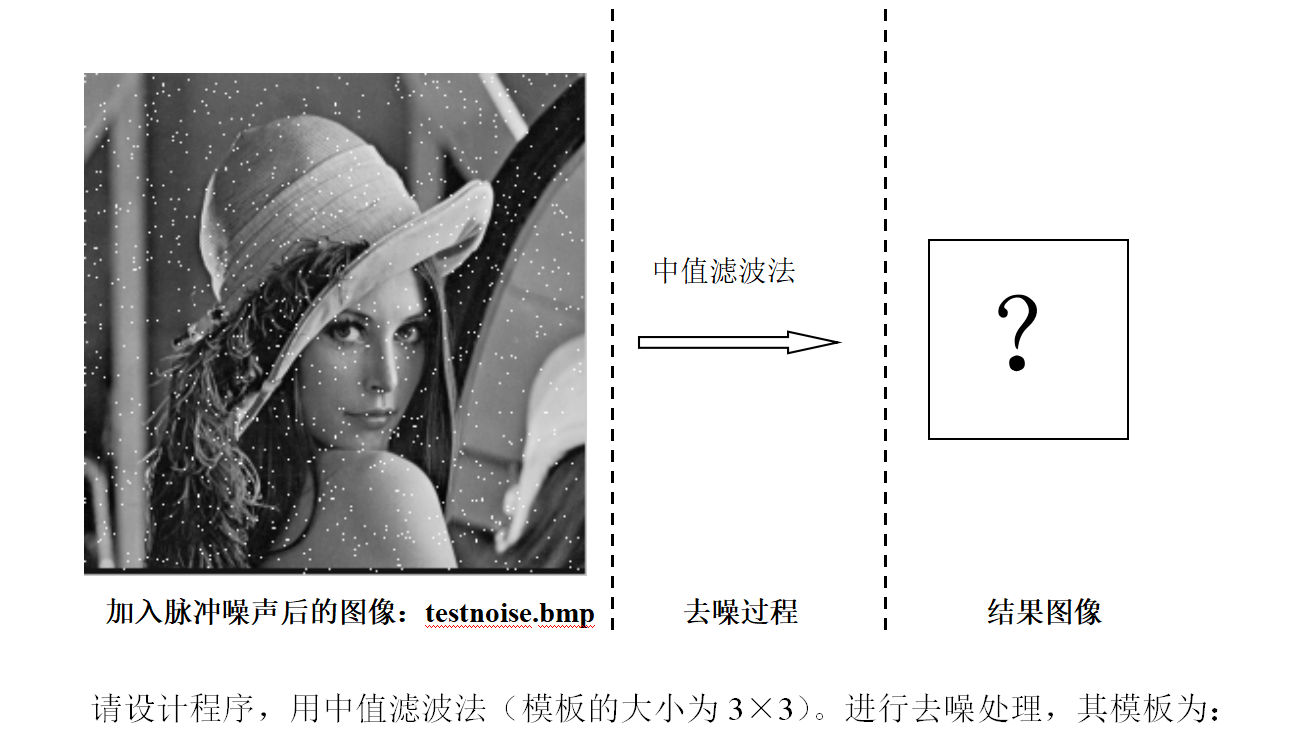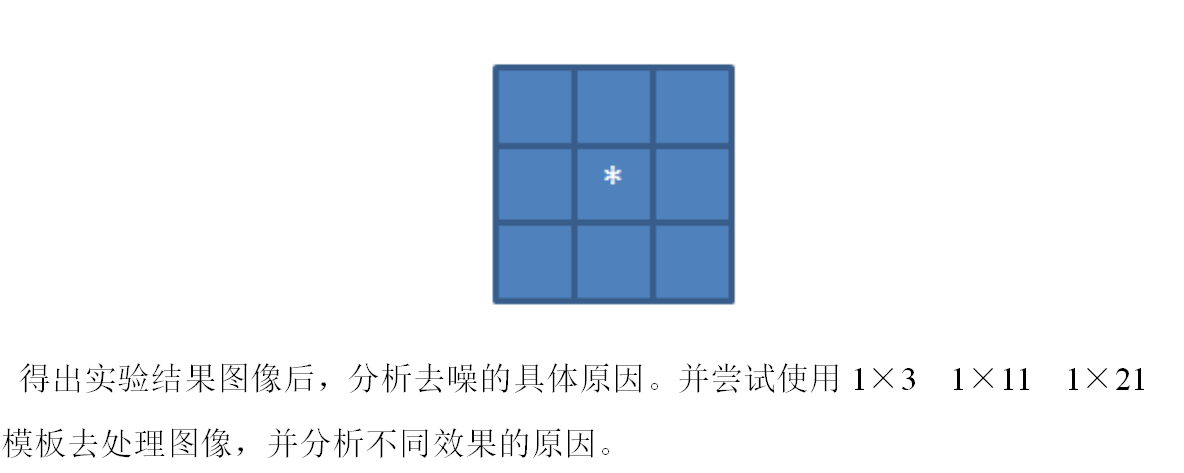• 中值滤波的处理过程是选择像素周围的一片模板大小的区域，计算这个区域的每个位置的像素，然后取这个区域的像素的中位数作为这个点的像素大小，然后输出，当然为了方便应该尽量选择奇数

3_3.c

#include <stdio.h>
#include <stdlib.h>
#include <memory.h>
#include "hdr.h"
struct bmphdr *hdr;
unsigned char *bitmap, *to;
char buf;
int di = {0, 0, 1, 1, 1, -1, -1, -1};//八个方向
int dj = {1, -1, 0, 1, -1, 0, 1, -1};
unsigned char tmp;
inline int cmp(const void *a, const void *b){
unsigned char *t1, *t2;
t1 = (unsigned char *)a;
t2 = (unsigned char *)b;
if(*t1 < *t2) return -1;
else if(*t1 > *t2) return 1;
else return 0;
}
int main(int argc, char *argv[]){
int i, j, k, nr_pixels;//定义整数i,j用于函数循环
//nr_pixels为图像中像素的个数
FILE *fp, *fpnew;
unsigned pj, px;
argc = 3;
argv = "testnoise.bmp";
argv = "3_3.bmp";
int ii, jj;
if(argc != 3){
printf("Usage: %s <file_from> <file_to>\n", argv);
exit(1);
}
if(!hdr) exit(1);
fp = fopen(argv, "rb");
if(!fp){
printf("File open error!\n");
exit(1);
}
fseek(fp, hdr->offset, SEEK_SET);
nr_pixels = hdr->width * hdr->height;
bitmap = malloc(nr_pixels);
fclose(fp);
to = malloc(nr_pixels);
for(i=0;i<hdr->height;i++){
for(j=0;j<hdr->width;j++){
if(i == 0 || i == hdr->height- 1|| j == 0 ||j == hdr->width - 1){
to[i * hdr->width + j] = bitmap[i * hdr->width + j];
}else{
for(k=0;k<8;k++){
ii = i + di[k];
jj = j + dj[k];
tmp[k] = bitmap[ii * hdr->width + jj];
}
tmp = bitmap[i * hdr->width + j];
qsort(tmp, 9, 1, cmp);
to[i * hdr->width + j] = tmp;
}
}
}
fpnew = fopen(argv, "wb+");
if(!fpnew){
printf("File create error!\n");
exit(1);
}
fwrite(hdr->signature, 2, 1,fpnew);
fwrite(&hdr->size, 4, 1, fpnew);
fwrite(hdr->reserved, 4, 1, fpnew);
fwrite(&hdr->offset, 4, 1, fpnew);
fwrite(&hdr->hdr_size, 4, 1, fpnew);
fwrite(&hdr->width, 4, 1, fpnew);
fwrite(&hdr->height, 4, 1, fpnew);
fwrite(&hdr->nr_planes, 2, 1, fpnew);
fwrite(&hdr->bits_per_pixel, 2, 1, fpnew);
fwrite(&hdr->compress_type, 4, 1, fpnew);
fwrite(&hdr->data_size, 4, 1, fpnew);
fwrite(&hdr->resol_hori, 4, 1, fpnew);
fwrite(&hdr->resol_vert, 4, 1, fpnew);
fwrite(&hdr->nr_colors, 4, 1, fpnew);
fwrite(&hdr->important_color, 4, 1, fpnew);
if (hdr->offset > 54) fwrite(hdr->info, (hdr->offset - 54), 1, fpnew);
//直方图均衡化的数据(bitmap)赋值
fwrite(to, nr_pixels, 1, fpnew);
fclose(fpnew);
free(hdr);
free(bitmap);
return 0;
}

hdr.h

#ifndef __HDR_H__
#define __HDR_H__

struct bmphdr {
char signature;//文件类型
int size;//位图大小
short reserved;//两个保留字
int offset;//位图偏移位置
int hdr_size;//头文件大小
int width;//位图宽度
int height;//位图高度
short nr_planes;//位平面数
short bits_per_pixel;//每像素的位数
int compress_type;//压缩类型
int data_size;//数据大小
int resol_hori;//水平
int resol_vert;//垂直
int nr_colors;
int important_color;
char info;
};

#endif

hdr.c

#include <stdio.h>
#include <memory.h>
#include "hdr.h"

{
FILE *fp;
struct bmphdr *hdr;

fp = fopen(filename, "rb");
if (!fp) {
printf("File open error or such file does not exist!\n");
return NULL;
}

hdr = (struct bmphdr *)malloc(sizeof(struct bmphdr));//分配空间
if (hdr->signature != 'B' || hdr->signature != 'M') {//如果打开文件的扩展名不是.bmp
printf("Not a bmp file!\n");
return NULL;
}
//读位图头文件信息
if (hdr->offset > 54)//获得位图有效信息，偏移量为54的时候读的才是位图第一个像素

fclose(fp);//关闭文件
return hdr;
}

处理结果如下• 如果想把模板改成 1 × 11 1\times11 ，那么就是1行11列，所以只需要把方向改一下即可，像这样
int dj = {-5, -4, -3, -2, -1, 0, 1, 2, 3, 4, 5};

注意数组大小也都要改好，当然这是在列上进行的改变，行不动，很简单，这样处理之后得到图像如下剩下 1 × 21 1\times21 等等一个道理

展开全文• opencv-11-中值滤波及自适应中值滤波 计算机视觉 opencv 算法 c++ qt5

开始之前 在上一篇我们实现了读取噪声图像, 然后 进行三种形式的均值滤波得到结果, 由于我们自己写的均值滤波未作边缘处理, 所以效果有一定的下降,...首先介绍了中值滤波的原理, 给出其实现思路,并根据思路实现了 C+...

开始之前

在上一篇我们实现了读取噪声图像, 然后 进行三种形式的均值滤波得到结果, 由于我们自己写的均值滤波未作边缘处理, 所以效果有一定的下降, 但是总体来说, 我们得到的结果能够说明我们的算法执行之后得到的图像噪声更低, 图像更清晰. 但是也会造成图像的模糊, 导致部分细节丢失. 在这一章中,我们介绍一下中值滤波及其实现

摘要

首先介绍了中值滤波的原理, 给出其实现思路,并根据思路实现了 C++ 的代码, 然后 同样测试 opencv 自带的中值滤波, 同样的测试图像, 得到对比结果, 分析代码的实现过程, .

正文

中值滤波原理

中值滤波(Media Filter)就是对于图像的每一个点计算其邻域窗口的像素序列中值, 可以表示为:
g ( x , y ) = m e i d a ( i , j ) ∈ S f ( i , j ) g(x,y) = meida_{(i,j) \in S}f(i,j)
核心就是将相应窗口内的像素值进行排列, 我们之前也说过, 我们选择的窗口为奇数尺寸, 所以我们能够保证窗口内的像素个数也是奇数个, 这样我们可以保证取得唯一的中值, 相应的设置为该点的目标值就行了.

C++ 实现中值滤波

我们来实现一下, 这方面还是能够找到不少结果的, 感觉这个博主写的还是很不错的,有兴趣的可以看下数字图像处理------中值滤波,还有图像处理之中值滤波介绍及C实现, 或者 中值滤波器（Median filter）特性及其实现, 这里我就不再造轮子了, 我们来看下 C++的实现
, 主要参考 第一篇文章, 可以看下效果

这里有一点点需要讨论的, 对于彩色图像的三个通道怎么处理, 自己的思路就是分成三个通道进行处理, 然后分别得到三个图之后进行合并三个通道, 得到结果图像. 查了下 目测大家都是这么做的, 可以看OpenCV 彩色图像的自适应中值滤波 C++彩色图像空间滤波（MATLAB） 这两篇文章, 思路都是一样的, 我们来实现一下.

//中值滤波：C++ 代码实现 // 处理单通道图像 // 参考 https://www.cnblogs.com/ranjiewen/p/5699395.html
cv::Mat medianFilterGray(const cv::Mat &src, int ksize = 3)
{
cv::Mat dst = src.clone();
//0. 准备：获取图片的宽，高和像素信息，
const int  num = ksize * ksize;
std::vector<uchar> pixel(num);

//相对于中心点，3*3领域中的点需要偏移的位置
int delta[3 * 3] = {
{ -1, -1 }, { -1, 0 }, { -1, 1 }, { 0, -1 }, { 0, 0 }, { 0, 1 }, { 1, -1 }, { 1, 0 }, {1, 1}
};
//1. 中值滤波，没有考虑边缘
for (int i = 1; i < src.rows - 1; ++i)
{
for (int j = 1; j < src.cols - 1; ++j)
{
//1.1 提取领域值 // 使用数组 这样处理 8邻域值 不适合更大窗口
for (int k = 0; k < num; ++k)
{
pixel[k] = src.at<uchar>(i+delta[k], j+ delta[k]);
}
//1.2 排序  // 使用自带的库及排序即可
std::sort(pixel.begin(), pixel.end());
//1.3 获取该中心点的值
dst.at<uchar>(i, j) = pixel[num / 2];
}
}
return dst;
}

思路还是那个思路, 不过在写的过程中, 我在想, 能不能直接处理彩色的图像呢, 对于彩色图像最麻烦的地方就是排序了, 我们没办法考虑颜色的高低值, 所以 那我们自定义一个比较函数应该就行了吧. 我们使用三个颜色的和值 做比较
这里使用了C++ 的sort 自定义函数的方法, 这边采用的比较函数的方式, 还有别的方式实现两个元素的比较, 可以参考c++中vector自定义排序的问题

// 自定义两个像素的比较函数,  // 使用和值 排序
bool comp(const cv::Vec3b &p1, const cv::Vec3b &p2)
{
return (p1 + p1 + p1) < (p2 + p2 + p2);
}
// 尝试彩色图像, 中值排序使用三个通道的和排序
cv::Mat medianFilterColor(const cv::Mat &src, int ksize = 3)
{
cv::Mat dst = src.clone();
//0. 准备：获取图片的宽，高和像素信息，
const int  num = ksize * ksize;
std::vector<cv::Vec3b> pixel(num);

//相对于中心点，3*3领域中的点需要偏移的位置
int delta[3 * 3] = {
{ -1, -1 }, { -1, 0 }, { -1, 1 }, { 0, -1 }, { 0, 0 }, { 0, 1 }, { 1, -1 }, { 1, 0 }, {1, 1}
};
//1. 中值滤波，没有考虑边缘
for (int i = 1; i < src.rows - 1; ++i)
{
for (int j = 1; j < src.cols - 1; ++j)
{
//1.1 提取领域值 // 使用数组 这样处理 8邻域值 不适合更大窗口
for (int k = 0; k < num; ++k)
{
pixel[k] = src.at<cv::Vec3b>(i + delta[k], j + delta[k]);
}
//1.2 排序  // 使用自定义的排序函数排序彩色图像
std::sort(pixel.begin(),pixel.end(),comp);
//1.3 获取该中心点的值
dst.at<cv::Vec3b>(i, j) = pixel[num / 2];
}
}
return dst;
}

opencv 中值滤波

这里还是之前的方法, 一样的接口, 实现起来很简单, opencv 提供的 函数还是很丰富的, 很厉害

// opencv 中值滤波
cv::Mat mediaFilterDefault(const cv::Mat &src, int ksize = 3)
{
cv::Mat dst;
cv::medianBlur(src, dst, ksize);
return dst;
}

中值滤波算法对比

我们这里就跟之前均值算法的计算很相似了, 我们已经写了三种算法的实现, 然后测试就好了, 趁着功夫, 将上一章一直重复的两个图比较并输出参数的部分写成了一个函数

// 对比两个图像 然后输出 参数信息
QString compareImages(const cv::Mat &I1,
const cv::Mat &I2,
const QString str = "noise",
const QString str_temp = "image-%1: psnr:%2, mssim: B:%3 G:%4 R:%5")
{
double psnr_ = getPSNR(I1, I2);
cv::Scalar mssim_ = getMSSIM(I1, I2);

// 根据 输出模板 生成参数信息
QString res_str = str_temp.arg(str)
.arg(psnr_)
.arg(mssim_.val)
.arg(mssim_.val)
.arg(mssim_.val);

return res_str;
// cv::imwrite(IMAGE_DIR + "dst_" + std::to_string(i + 1) + ".png", dst[i]);
}

没什么难度, 就是用来拼接一个字符串, 用来显示在界面上, 或者 输出输出来,

这样的我们就能很容易的去写测试的函数了, 三种方法依次去实现, 比较麻烦的是第一种, 需要将彩色图像分成三个通道的灰度图像, 然后分别进行中值滤波, 最后合并结果,得到结果图像.

void MainWindow::testFunc2(void)
{
// 测试 中值 滤波 三种方式的不同
const int TEST = 1; // 使用统一的图进行测试 暂时使用 高 椒盐噪声图像
QString res_str;

// 噪声图像的参数值
res_str = compareImages(gSrcImg, gNoiseImg[TEST]);
ui->pt_log->appendPlainText(res_str);

cv::Mat test_img = gNoiseImg[TEST];

cv::Mat dst;

// 测试 中值滤波 拆分三个通道进行中值滤波然后合并图像
std::vector<cv::Mat> bgr(3);
cv::split(test_img, bgr);
bgr = medianFilterGray(bgr);
bgr = medianFilterGray(bgr);
bgr = medianFilterGray(bgr);

cv::merge(bgr, dst);     // 第一种方式
dst = medianFilterColor(test_img);   // 第二种 彩色直接 计算中值滤波
dst = mediaFilterDefault(test_img);  // opencv 实现 中值滤波

// 分别计算三种方式得到的滤波的效果 (结果图与 原始图比较)
for(int i=0;i<3;i++)
{
res_str = compareImages(gSrcImg, dst[i]);
// 噪声的参数值
ui->pt_log->appendPlainText(res_str);

cv::imwrite(IMAGE_DIR + "dst_media_" + std::to_string(i+1)+".png",dst[i]);
}
}

我们仍然选择高椒盐噪声图像用于测试, 先看下结果, 分别对应噪声图的参数, 以及三种方法进行的参数结果.
第三行的结果就是我们进行自定义排序的图像处理,

image-noise: psnr:19.4727, mssim: B:0.353134 G:0.383638 R:0.629353
image-noise: psnr:33.3725, mssim: B:0.896859 G:0.915976 R:0.912563
image-noise: psnr:31.2668, mssim: B:0.866162 G:0.901717 R:0.879337
image-noise: psnr:34.3125, mssim: B:0.902338 G:0.921419 R:0.91531

我们看一下结果图像, 原始图像可以看 https://gitee.com/schen00/BlogImage/raw/master/image/1588468343599.png 这里,

gitee 限制了 1M 以上的图的显示, 所以有需要的去看这个就好.

最近一直用的图拼接使用的 做好图 在线拼接图片 主要是懒得自己写了, http://www.zuohaotu.com/image-merge.aspx 链接在这里了 有需要自取这里的第一副图是噪声图像, 第二副是我们拆分通道处理后拼接起来了的, 没有处理边缘的细节问题, 第三章图就是我们进行自定义中值排序得到的图, 部分点处理不掉 甚至还复制了出来, 不过整体效果还是不错的, 第四章图就是opencv 自带的中值滤波的处理.

中值滤波算法优化

类似均值滤波, 处理的时候考虑变化了的边界就好了, 那中值滤波怎么优化呢, 感觉这一块做的人还挺多, 中值滤波的优化主要是使用自适应中值滤波, 和在中值滤波的方法上进行加速运算,

自适应中值滤波

可以参考自适应中值滤波及实现, 我感觉介绍的还是比较详细的, 主要的思路就是如果噪声比较严重时, 窗口获取到的中值可能是噪声值, 这时候增大窗口, 然后重新进行中值滤波,直到找到比较符合的中值.
引用他给出的部分叙述

在自适应中值滤波算法中，A步骤里面会先判断是否满足 Z m i n < Z m e d < Z m a x Z m i n < Z m e d < Z m a x Zmin<Zmed<ZmaxZmin<Zmed<Zmax 。这一步骤实质是判断当前区域的中值点是否是噪声点，通常来说是满足 Z m i n < Z m e d < Z m a x Z m i n < Z m e d < Z m a x Zmin<Zmed<ZmaxZmin<Zmed<Zmax 这个条件的，此时中值点不是噪声点，跳转到B；考虑一些特殊情况，如果 Z m e d = Z m i n Z m e d = Z m i n 或 者 Z m e d = Z m a x Z m e d = Z m a x Zmed=ZminZmed=Zmin或者Zmed=ZmaxZmed=Zmax ，则认为是噪声点，应该扩大窗口尺寸，在一个更大的范围内寻找一个合适的非噪声点，随后再跳转到B，否则输出的中值点是噪声点；
接下来考虑跳转到B之后的情况：判断中心点的像素值是否是噪声点，判断条件为 Z m i n < Z x y < Z m a x Z m i n < Z x y < Z m a x Zmin<Zxy<ZmaxZmin<Zxy<Zmax ，原理同上，因为如果 Z x y = Z m i n Z x y = Z m i n Zxy=ZminZxy=Zmin 或者 Z x y = Z m a x Z x y = Z m a x Zxy=ZmaxZxy=Zmax ，则认为是噪声点。如果不是噪声点，我们可以保留当前像素点的灰度值；如果是噪声点，则使用中值替代原始灰度值，滤去噪声。

同样的, 图像处理基础(2)：自适应中值滤波器(基于OpenCV实现), 这篇文章写的更好一点, 并给出了 opencv 的实现代码, 我们来看一下

// 自适应中值滤波窗口实现  // 图像 计算座标, 窗口尺寸和 最大尺寸
uchar adaptiveProcess(const Mat &im, int row, int col, int kernelSize, int maxSize)
{
std::vector<uchar> pixels;
for (int a = -kernelSize / 2; a <= kernelSize / 2; a++)
for (int b = -kernelSize / 2; b <= kernelSize / 2; b++)
{
pixels.push_back(im.at<uchar>(row + a, col + b));
}
sort(pixels.begin(), pixels.end());
auto min = pixels;
auto max = pixels[kernelSize * kernelSize - 1];
auto med = pixels[kernelSize * kernelSize / 2];
auto zxy = im.at<uchar>(row, col);
if (med > min && med < max)
{
// to B
if (zxy > min && zxy < max)
return zxy;
else
return med;
}
else
{
kernelSize += 2;
if (kernelSize <= maxSize)
return adaptiveProcess(im, row, col, kernelSize, maxSize); // 增大窗口尺寸，继续A过程。
else
return med;
}
}
// 自适应均值滤波
cv::Mat adaptiveMediaFilter(const cv::Mat &src, int ksize = 3)
{
int minSize = 3; // 滤波器窗口的起始尺寸
int maxSize = 7; // 滤波器窗口的最大尺寸
cv::Mat dst;
// 扩展图像的边界
cv::copyMakeBorder(src, dst, maxSize / 2, maxSize / 2, maxSize / 2, maxSize / 2, cv::BorderTypes::BORDER_REFLECT);
// 图像循环
for (int j = maxSize / 2; j < dst.rows - maxSize / 2; j++)
{
for (int i = maxSize / 2; i < dst.cols * dst.channels() - maxSize / 2; i++)
{
dst.at<uchar>(j, i) = adaptiveProcess(dst, j, i, minSize, maxSize);
}
}
cv::Rect r = cv::Rect(cv::Point(maxSize / 2, maxSize / 2), cv::Point(dst.rows-maxSize / 2, dst.rows-maxSize / 2));
cv::Mat res = dst(r);
return res;
}

我们这里还是使用的分离三个通道然后进行自适应均值滤波, 参数就使用默认的3, 最大窗口设为7, 我们测试还是跑的之前的高椒盐噪声图像, 下面给出的最后一行就是我们使用自适应中值滤波得到的结果, 至少从 psnr 的参数上我们能看到图像质量的提升, 我们给出图像结果, 肉眼上能看出稍微一点的区别, 对比之前的已经完全不存在白点了, 图像已经比较接近真实图像了…

// 拆分三个通道 计算自适应中值滤波
cv::split(test_img, bgr);
for (int i = 0; i < 3; i++)
cv::merge(bgr, dst);

image-noise: psnr:19.4727, mssim: B:0.353134 G:0.383638 R:0.629353
image-noise: psnr:33.3725, mssim: B:0.896859 G:0.915976 R:0.912563
image-noise: psnr:31.2655, mssim: B:0.86636 G:0.901517 R:0.879384
image-noise: psnr:34.3125, mssim: B:0.902338 G:0.921419 R:0.91531
image-noise: psnr:37.4024, mssim: B:0.946158 G:0.958146 R:0.953884中值滤波计算加速

由于中值滤波无论多大的窗口都是用来将窗口内的像素进行排序, 这里的优化有两个方向 一个是窗口的优化, 一个计算的加速,

我真的 imageshop 的这篇文章 任意半径中值滤波（扩展至百分比滤波器）O(1)时间复杂度算法的原理、实现及效果。
已经写的比较完全了, 我都不想在写了,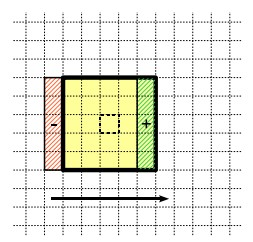再从中值滤波的快速算法 偷一张图,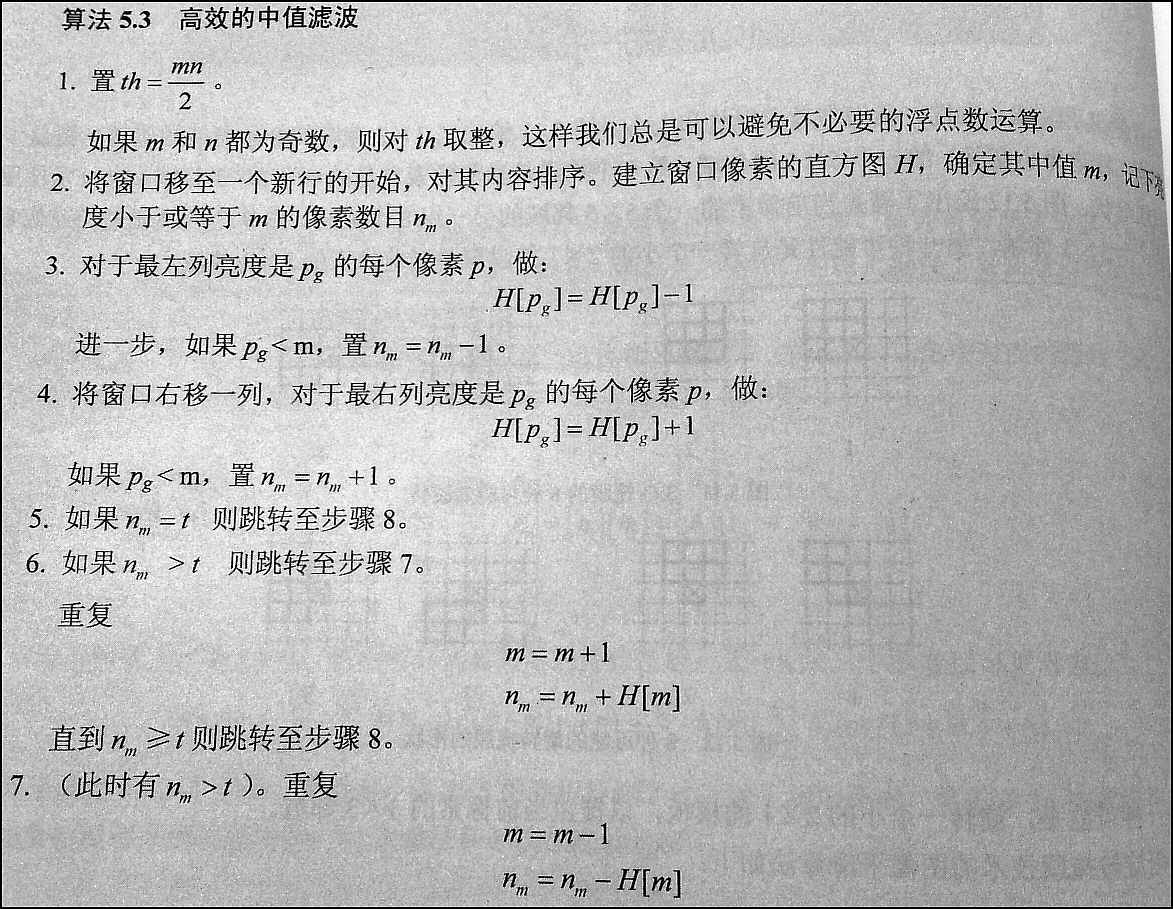感兴趣的可以看一下的链接
OpenCV源码分析（四）：中值滤波 这里详细介绍了 opencv 中怎么实现的 中值滤波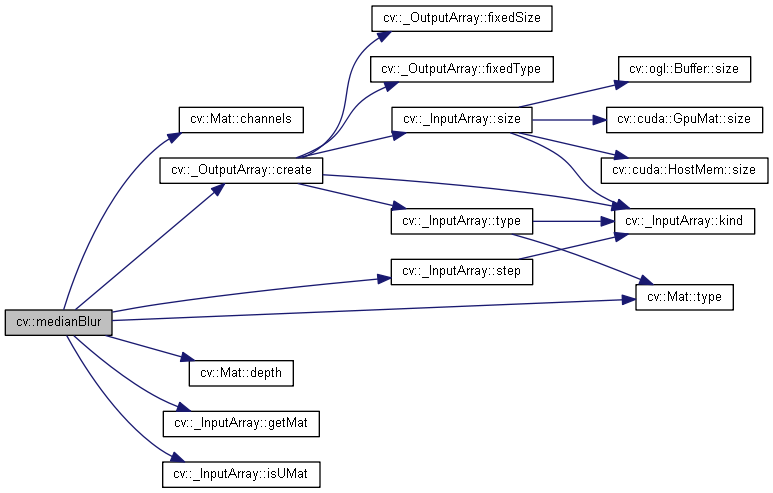总结

算是从中值滤波的基础上做了一个开始, 介绍了一下中值滤波的原理, 然后根据原理使用C++ 进行了实现, 之后再进行 opencv 的实现, 然后我们根据之前的程序上加入了中值滤波的实现效果, 最后在中值滤波的基础上进行优化, 做了自适应中值滤波的实现,测试发现结果还要更好, 最后我稍微提了一下中值滤波的优化加速, 这一块做的很多, 可以去参考里面去找, 算是完成了中值滤波的章节, 如果这里搞懂了我再来完善这一章节…

参考

1. 《绘制函数调用图（call graph）（4）：doxygen + graphviz_运维_许振坪的专栏-CSDN博客》. 见于 2020年5月2日. https://blog.csdn.net/benkaoya/article/details/79763668.
2. 《任意半径中值滤波（扩展至百分比滤波器）O(1)时间复杂度算法的原理、实现及效果。 - Imageshop - 博客园》. 见于 2020年5月3日. https://www.cnblogs.com/Imageshop/archive/2013/04/26/3045672.html.
3. 《数字图像处理------中值滤波 - ranjiewen - 博客园》. 见于 2020年5月2日. https://www.cnblogs.com/ranjiewen/p/5699395.html.
4. 《【算法随记三】小半径中值模糊的急速实现（16MB图7.5ms实现） + Photoshop中蒙尘和划痕算法解读。 - Imageshop - 博客园》. 见于 2020年5月3日. https://www.cnblogs.com/Imageshop/p/11087804.html.
5. 《图像处理基础(2)：自适应中值滤波器(基于OpenCV实现) - Brook_icv - 博客园》. 见于 2020年5月3日. https://www.cnblogs.com/wangguchangqing/p/6379646.html.
6. 《图像处理之原理 - 中值滤波 - tanfy - 博客园》. 见于 2020年5月2日. https://www.cnblogs.com/tanfy/p/median_filter.html.
7. 《图像处理之中值滤波介绍及C实现 - 淇淇宝贝 - 博客园》. 见于 2020年5月2日. https://www.cnblogs.com/qiqibaby/p/5281743.html.
8. 《中值滤波的快速算法_网络_LinJM-机器视觉-CSDN博客》. 见于 2020年5月3日. https://blog.csdn.net/linj_m/article/details/35780163.
9. 《中值滤波器》. 收入 维基百科，自由的百科全书, 2017年9月8日. https://zh.wikipedia.org/w/index.php?title=%E4%B8%AD%E5%80%BC%E6%BB%A4%E6%B3%A2%E5%99%A8&oldid=46098815.
10. 《中值滤波器（Median filter）特性及其实现_人工智能_Ivan 的专栏-CSDN博客》. 见于 2020年5月2日. https://blog.csdn.net/liyuanbhu/article/details/48502005.
11. 《自适应中值滤波及实现_人工智能_hongbin_xu的博客-CSDN博客》. 见于 2020年5月3日. https://blog.csdn.net/hongbin_xu/article/details/79780967.
12. GitHub. 《ARM-Software/ComputeLibrary》. 见于 2020年5月3日. https://github.com/ARM-software/ComputeLibrary.
13. 《c++中vector自定义排序的问题_C/C++_Stone_Sky-CSDN博客》. 见于 2020年5月2日. https://blog.csdn.net/aastoneaa/article/details/8471722.
14. 《OpenCV 彩色图像的自适应中值滤波 C++_人工智能_cyf15238622067的博客-CSDN博客》. 见于 2020年5月3日. https://blog.csdn.net/cyf15238622067/article/details/88718615.
15. 《‪opencv: ‪Image Filtering》. 见于 2020年5月3日. http://schen.xyz:89/opencv/d4/d86/group__imgproc__filter.html#gad7c87bbc46b97e7eafa71357916ab568.
16. 知乎专栏. 《OpenCV图像处理专栏九 | 基于直方图的快速中值滤波算法》. 见于 2020年5月3日. https://zhuanlan.zhihu.com/p/98092747.
17. 简书. 《OpenCV源码分析（四）：中值滤波》. 见于 2020年5月2日. https://www.jianshu.com/p/eb0b856286f2.
展开全文HugoChen_cs 2020-05-03 11:56:29
• 615KB weixin_38681719 2020-05-27 14:16:18
•5星
326KB k342242175 2012-06-02 13:39:23
• 图像处理——中值滤波 图像处理 python 计算机视觉

lixiao0314 2021-10-25 11:13:56
• 高斯滤波 中值滤波 均值滤波 图像处理

zml194849 2021-02-25 10:27:32
• 数字图像处理——中值滤波及其改进算法 算法 中值滤波

qq_21842097 2021-08-27 17:35:28
• weixin_39665507 2021-04-18 10:44:28
• 中点和中值滤波的区别_【从零学习OpenCV 4】中值滤波 中点和中值滤波的区别

weixin_39618339 2020-10-22 00:56:30
• JAVA空间滤波——中值滤波 数字图像处理 JAVA 空间滤波 中值滤波算法

weixin_43524214 2019-10-09 16:31:13
• weixin_42113754 2021-04-29 10:49:49
• 超入门级-基于中值滤波处理ECG信号的基线漂移-Python-MIT-BIH数据集 python 深度学习 机器学习

chrnhao 2021-09-22 18:24:18
• 图像平滑处理-中值滤波 图像平滑处理 中值滤波 图像处理 计算机视觉处理

Monster_H7 2018-11-06 15:57:18
• 3x3中值滤波怎么计算过程_单片机数字滤波算法，看这篇 低通滤波器算法实现 单片机歌曲代码大全

weixin_39761558 2020-11-21 01:28:30
• weixin_43329242 2020-09-21 20:00:10
• weixin_36251052 2021-05-19 16:34:54
• FPGA verilog HDL实现中值滤波 中值滤波 fpga

qq_40310273 2020-05-24 14:35:01
• 数字图像处理:图像平滑 (均值滤波、中值滤波和高斯滤波) 图像 滤波 数字图像处理

ShaoDu 2019-07-19 09:29:04
• 数字图像处理与Python实现-图像滤波-自适应中值滤波 图像滤波 图像处理 图像降噪 计算机视觉 机器视觉

wujuxKkoolerter 2021-03-26 17:15:50
• weixin_29792607 2021-05-23 04:29:24...